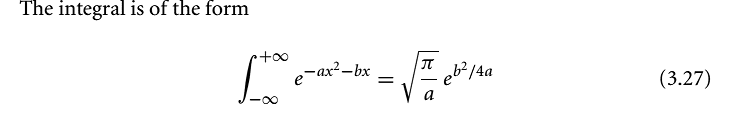# Initial momentum amplitude to wave function

## Homework Statement

http://www.whoisntdavidrinaldi.com/Untitled.png [Broken]

## The Attempt at a Solution

So I had to use this initial momentum and multiply it by a plane wave (sure you are aware of the exp[i(px+p^2(t)/2m)\hbar] )
setting p-p_0 to q and then coupling all terms together to get an integral of the form where one could complete the square the problem here is the extra q, which seems to force me to to integration by parts. The problem is I can set u=q but if dv is my exponential term what is v? I've never seen how to

\int_{-\infty}^\infty dq(q)exp[-q^2(\frac{\alpha^2}{2}+\frac{it}{2m\hbar})+ q(\frac{ix}{\hbar}+\frac{ipt}{2m\hbar})

crud. I'm not sure how to post latex here. I guess this is my second question. To see the above the link is here www.whoisntdavidrinaldi.com\help1.pdf [Broken]

Last edited by a moderator:

Simon Bridge
Homework Helper
##\small \psi(x)## is the fourier transform of ##\small \phi(p)## - time-evolve - use the derivation for eq3.61 to guide your algebra.

##\small \LaTeX2e## is posted between double-hash marks for inline equations and double-dollar signs for display math. Use the "quote" button (bottom left of this post) to see the following example:
$$\int \phi(p)=\sqrt{\frac{2\alpha^3}{\sqrt{\pi}}}(p-p_0)e^{-\alpha^2(p-p_0)^2/2}$$

exp[i(px+p^2(t)/2m)\hbar] (a bit of confusion about brackets there?) comes out as: $$e^{-i(px+p^2t/2m)\hbar }$$

The problem is I can set u=q but if dv is my exponential term what is v? I've never seen how to

You are trying to do:
$$\int_{-\infty}^\infty q\exp\left[-q^2(\frac{\alpha^2}{2}+\frac{it}{2m\hbar})+ q(\frac{ix}{\hbar}+\frac{ipt}{2m\hbar})\right ]\; dq$$ ... I tidied up your latex a bit.

Integrals of form: ##\int \exp [x^2]dx## have no analytic solution.

Last edited:
$$\int_{-\infty}^\infty q\exp\left[-q^2(\frac{\alpha^2}{2}+\frac{it}{2m\hbar})+ q(\frac{ix}{\hbar}+\frac{ipt}{2m\hbar})\right ]\; dq$$ ... I tidied up your latex a bit.

Integrals of form: ##\int \exp [x^2]dx## have no analytic solution.

if we look at my first equation, and forget about the extra q lying around, we get something of the form

##\int_{-\infty}^\infty \exp [-x^2+x]dx##

or $$\int_{-\infty}^\infty \exp [-x^2-x]dx$$

do have solutions..it's just that this new q makes me need to use integration by parts as well.

Simon Bridge
Homework Helper
if we look at my first equation, and forget about the extra q lying around, we get something of the form

##\int_{-\infty}^\infty \exp [-x^2+x]dx##

or $$\int_{-\infty}^\infty \exp [-x^2-x]dx$$

do have solutions..it's just that this new q makes me need to use integration by parts as well.
... well $$\int\exp[ax-bx^2]dx = \frac{\sqrt{\pi}}{2\sqrt{b}}\exp \left[\frac{a^2}{4b}\right]\text{erf}\left[\frac{2bx-a}{2\sqrt{b}}\right]+c$$ ... but you have an integral of complex exponential so you could try to express is as a sum of sines and cosines.

I am confusedSimon Bridge
Homework Helper
That differs from what you wrote in post #1... you have bx-ax^2 in the exponent.

$$\int xe^{-ax^2-bx} dx = \frac{b\sqrt{pi}}{4a^{3/2}}e^{\frac{b^2}{4a}}\text{erf}\bigg(\frac{2ax+b}{2\sqrt{a}}\bigg)-\frac{1}{2a}e^{-x(ax+b)}+c$$

I've still got the nagging feeling you should also separate real and imaginary parts - especially considering you'll end up taking the complex conjugate after.

Last edited:
Why don’t you complete the square in the integral?
let a = α2/2 + ipt/2mhbar
b = ix/hbar + ipt/2mhbar
e-(a + b*b/4a*a)∫qe(b/2a-q)*(b/2a-q)dq

and making the substitution q’ = b/2a-q
I get
e-(a + b*b/4a*a)[(b/2a)e-q’*q’-√(π/4)erf(√q’) ]

--sorry about the notation. I don't know how to type the square of an exponential argument,

Last edited:
Simon Bridge